# Loops in Java

#### View more Tutorials: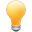Follow us on our fanpages to receive notifications every time there are new articles.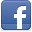Facebook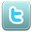Twitter

## 1- Loops in Java

In computer programming, a loop is used regularly, and the purpose is to execute a program snippet several times. The Java supports 3 different kinds of loops:
1. for loop
2. while loop
3. do-while loop
The for loop has 2 variants:
1. Common for loop.
2. For-each loop (Introduced from Java 5 version ).
Statements can be used in a loop:
• break
• continue.

## 2- for Loop

Syntax:
** for **
``````
// start_value: Start Value
// end_value: End Value
// increment_number: Added value after each iteration.
for ( start_value; end_value; increment_number ) {
// Do something here
}
``````
Consider an illustration:
ForLoopExample1.java
``````
package org.o7planning.tutorial.javabasic.loop;

public class ForLoopExample1 {

public static void main(String[] args) {

// Declare a variable 'step', step in loop
int step = 1;

// Declare a variable value with the start value is 0
// After each iteration, value will increase 3
// And the loop will end when the value greater than or equal to 10
for (int value = 0; value < 10; value = value + 3) {

System.out.println("Step =" + step + "  value = " + value);

// Increase 1
step = step + 1;

}

}

}
``````
Results of running the ForLoopExample1 class:
``````
Step =1  value = 0
Step =2  value = 3
Step =3  value = 6
Step =4  value = 9
``````
See another example, sum the numbers from 1 to 100:
ForLoopExample2.java
``````
package org.o7planning.tutorial.javabasic.loop;

public class ForLoopExample2 {

// This is an example to sum the numbers from 1 to 100,
// and print out the results to the console.
public static void main(String[] args) {

// Declare a variable
int total = 0;

// Declare a variable value
// Initial value is 1
// After each iteration increases the 'value' by adding 1
// Note: value++ equivalent to the statement: value = value + 1;
// value-- equivalent to the statement: value = value - 1;
for (int value = 1; value <= 100; value++) {

// Increase 'total' by adding 'value'.
total = total + value;
}

System.out.println("Total = " + total);

}

}
``````
Result:
``````
Total = 5050
``````

## 3- for-each loop

The for-each loop is introduced into Java from version 5. As a loop used to traverse an array or a collection, it will move from the first element to the last element of the array or collection respectively.
See more:
Syntax:
``````
// arrayOrCollection: Array or Collection
for (Type variable: arrayOrCollection)  {
// Code ...
}
``````
Example:
ForeachExample.java
``````
package org.o7planning.tutorial.javabasic.loop;

public class ForeachExample {

public static void main(String[] args) {

// Declare an array of String.
String[] fruits = new String[] { "Apple", "Apricot", "Banana" };

// The for-each loop to traverse array.
for (String fruit : fruits) {

System.out.println(fruit);

}

}

}
``````
Run the example:
``````
Apple
Apricot
Banana
``````

## 4- while Loop

The while loop is used to execute a program snippet repeatedly, when a condition is still true. The while loop is usually used when iterations are not defined before (not fixed).
This is while loop structure:
** while **
``````
// While condition is true then do something.
while ( condition ) {
// Do something here...
}
``````
See illustration
WhileExample1.java
``````
package org.o7planning.tutorial.javabasic.loop;

public class WhileExampe1 {

public static void main(String[] args) {

int value = 3;

// While the 'value' is still less than 10, the loop still works.
while (value < 10) {

System.out.println("Value = " + value);

// Increase 'value' by adding 2
value = value + 2;
}
}

}
``````

## 5- do-while Loop

The do-while loop is used to execute a section of program many times.The characteristics of the do-while is that the block of statement is always executed at least once. After each iteration, the program will check the condition, if the condition is still correct, the statement block will be executed again.
This is the structure do-while loop:
** do while **
``````
// The do-while loop performs at least one iteration.
// And while the condition is true, it continues to work
do {
// Do something here.
}
while (condition);
``````
Example:
DoWhileExample1.java
``````
package org.o7planning.tutorial.javabasic.loop;

public class DoWhileExample1 {

public static void main(String[] args) {

int value = 3;

// do-while loop will execute at least once
do {

System.out.println("Value = " + value);

// Increase by 3
value = value + 3;

} while (value < 10);

}
}
``````
Result:
``````
Value = 3
Value = 6
Value = 9
``````

## 6- The break statement in loop

The break statement can appear in a loop. It is the statement that help the program exit the loop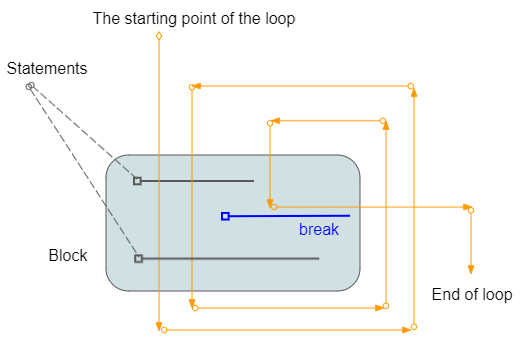Example:
LoopBreakExample.java
``````
package org.o7planning.tutorial.javabasic.loop;

public class LoopBreakExample {

public static void main(String[] args) {

System.out.println("Break example");

int x = 2;

while (x < 15) {

System.out.println("----------------------\n");
System.out.println("x = " + x);

// If x = 5 then exit the loop.
if (x == 5) {
break;
}

x++;
System.out.println("x after ++ = " + x);

}

System.out.println("Done!");

}

}
``````
Running the example:
``````
Break example
----------------------

x = 2
x after ++ = 3
----------------------

x = 3
x after ++ = 4
----------------------

x = 4
x after ++ = 5
----------------------

x = 5
Done!
``````

## 7- The continue statement in a loop

The continue statement can appear in a loop. When encountering the continue statement, the program will ignore the statement lines within the block and at the bottom of the continue and start an new iteration (If the condition is still correct).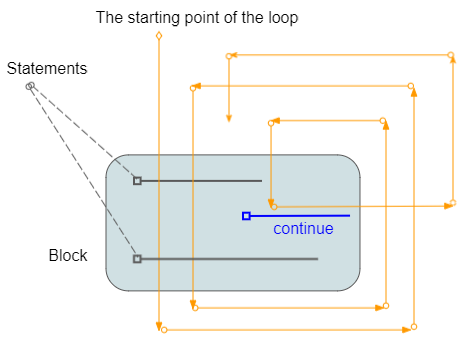Example:
LoopContinueExample.java
``````
package org.o7planning.tutorial.javabasic.loop;

public class LoopContinueExample {

public static void main(String[] args) {

System.out.println("Continue example");

int x = 2;

while (x < 7) {

System.out.println("----------------------\n");
System.out.println("x = " + x);

// x = x + 1;
x++;

// % operator is used for calculating remainder.
// If x is even, then ignore the command line below of 'continue',
// and start new iteration (if condition still true).
if (x % 2 == 0) {

continue;
}

System.out.println("x after ++ = " + x);

}

System.out.println("Done!");

}

}
``````
Run the example:
``````
Continue example
----------------------

x = 2
x after ++ = 3
----------------------

x = 3
----------------------

x = 4
x after ++ = 5
----------------------

x = 5
----------------------

x = 6
x after ++ = 7
Done!
``````

## 8- Labelled Loop

The Java allows you to stick a label to a loop. It is like you name a loop, which is useful when you use multiple nested loops in a program.
• You can use break labelX statement; to break a loop is attached labelX.
• You can use continue labelX statement; to continue a loop is attached​​​​​​​ labelX.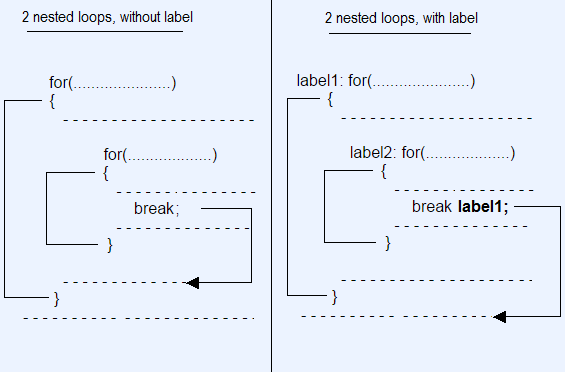Syntax:
** Labelled Loops **
``````
// for loop with Label.
label1: for( ... )  {

}

// while loop with Label.
label2: while ( ... )  {

}

// do-while loop with Label.
label3: do  {

} while ( ... );
``````
Example of using nested loops, labelled and labelled break statement.
LabelledLoopBreakExample.java
``````
package org.o7planning.tutorial.javabasic.loop;

public class LabelledLoopBreakExample {

public static void main(String[] args) {

System.out.println("Labelled Loop Break example");

int i = 0;

label1: while (i < 5) {

System.out.println("----------------------\n");
System.out.println("i = " + i);
i++;

label2: for (int j = 0; j < 3; j++) {

System.out.println("  --> " + j);
if (j > 0) {
// Exit the loop with label1.
break label1;
}
}

}

System.out.println("Done!");

}

}
``````
Run the example:
``````
Labelled Loop Break example
----------------------

i = 0
--> 0
--> 1
Done!
``````
Example of using nested labelled loops and labelled continue statement.
LabelledLoopContinueExample.java
``````
package org.o7planning.tutorial.javabasic.loop;

public class LabelledLoopContinueExample {

public static void main(String[] args) {

System.out.println("Labelled Loop Continue example");

int i = 0;

label1: while (i < 5) {
System.out.println("outer i= " + i);
i++;

label2: for (int j = 0; j < 3; j++) {
if (j > 0) {

continue label2;
}
if (i > 1) {

continue label1;
}
System.out.println("inner i= " + i + ", j= " + j);
}

}
}

}

``````
Run the example:
``````
Labelled Loop Continue example
outer i= 0
inner i= 1, j= 0
outer i= 1
outer i= 2
outer i= 3
outer i= 4
``````

#### View more Tutorials:

Maybe you are interested

These are online courses outside the o7planning website that we introduced, which may include free or discounted courses.

•Learn React JS from scratch: Create hands on projects
•The Complete Android App Development
•Complete guide to install Wordpress, PHP on Cloud,AWS or VPS
•Bootstrap 4 Single Page Website from Scratch
•Java Object-Oriented Programming : Build a Quiz Application
•AWS lambda for .NET C# developers
•Hibernate Object/relational mapping - ORM:Learn Popular Tool
•Flutter & Dart: A Complete Showcase Mobile App™
•Bootstrap 4.0 In Action: Build A Responsive Website
•C# Unity® and Swift 4 Masterclass: Make Games and Apps
•Learn Oracle 12c SQL : Kickstart kit for beginners
•Concepts of Object Oriented Programming with C++
•*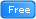* Java Database Connection: JDBC and MySQL
•Flutter ios&Android App With Firebase Backend (Project Base)
•Learn Android Programming From Scratch - Basics
•Java Hibernate Framework:Learn Object/Relational Mapping ORM
•Master AngularJS : Learn Angular JS From Scratch
•jQuery UI Ultimate:Design Amazing Interfaces Using jQuery UI
•Java JDBC with Oracle: Build a CRUD Application
•Learn Back-End Web Development: PHP and MySQL from scratch.
•Learn Swift Quick: IOS 11, Swift 4 and Xcode 9 Compatible!
•Master ReactJS: Learn React JS from Scratch
•Understanding JDBC with PostgreSQL (A step by step guide)
•Complete Step By Step Java For Testers